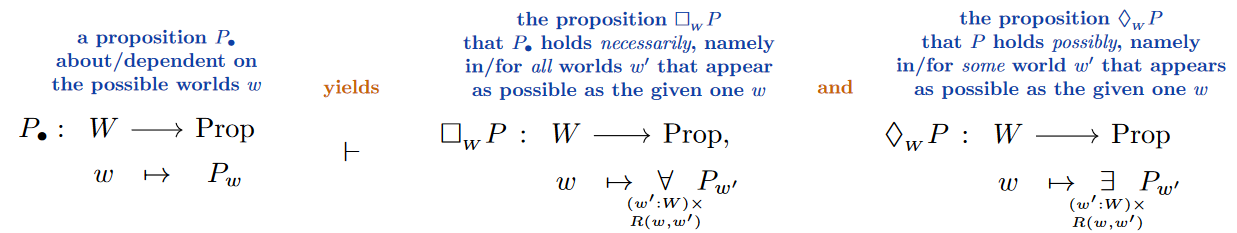# nLab Kripke frame

Contents

### Context

#### Modalities

Examples

This entry is about models of modal logic; for frames in the sense of geometric logic see instead there.

# Contents

## Idea

In formal logic, Kripke frames, after Kripke (1959), (1963), serve as the contextual substrate of set-theoretic semantics/models for modal logics (called geometric models as opposed to algebraic models).

In itself, a Kripke frame is just

• an inhabited set $W$

often called the set of possible worlds (or the set of nodes or whatnot)

• equipped with an indexed set of binary relations $R_i W \times W \to Prop$, one for each modal operator $\Box_i$ in the given modal logic

called the accessiblity or transition relations between worlds, or similar, where $R_i (w,w')$ is interpreted as: “To agent $i$ in world $w$, world $w'$ appears (just as well) possible.”

Hence a Kripke frame is a concept with an attitude, namely the attidute to serve as a model for a modal logic – the idea is to interpret:

1. the elements of $W$ as the “possible worlds” about which the propositions in the modal logic make statements,

2. the relation $R_i(w,w')$ as asserting that it makes sense to reason about world $w'$ at world $w$.

Namely a model of the modal logic based on such a Kripke frame will interpret

• each proposition $P$ of the logic as a $W$-dependent proposition $P \colon W \to Prop$,

• each modal proposition $\Box_i P$ as the $W$-dependent proposition which at $w \in W$ asserts that “$P$ holds at all those $w'$ that are in $i$th relation to $W$”, hence “$P$ holds in all worlds $w'$ that $i$ deems to be just as possible as $w$”; formally (eg. Blackburn & van Benthem (2007) p. 10):(Here we are writing — following notation for subtypes$P \,\colon\,W \to Prop$ for a $W$-dependent proposition, equivalently a proposition about the elements of $W$, equivalently the subset of $W$ where the proposition holds.)

The transparent case of S5 logic. Specifically, for the case of S5 modal logic with a single comonadic modal operator $\Box$, a Kripke frame (originally considered in Kripke 1963) is an

• inhabited set$\;$ $W$

equipped with a single

which means that $W$ decomposes as the disjoint union of its equivalence classes, these being the fibers of the quotient coprojection

(1)$W \xrightarrow{\;\; p_W \;\;} W/R \,.$

The simple case necessity logic. In the further special case there is just a single such class (hence that “all possible worlds are related”, originally considered by Kripke 1959) this becomes

(2)$W \xrightarrow{\;\; p_W \;\;} \ast$

so that in models based on such a frame the interpretation of the modal operator $\Box$ is that of necessity: $\Box P$ now asserts, independently of the world that it is evaluated on, that $P$ holds at all $w \in W$, hence that $P$ holds necessarily in the sense of: no matter which world.

The general case of Kripke frames is really just this basic idea with some variations added. For instance the general case (1) of S5 modal logic is just a $W/R$-indexed disjoint union of this basic situation.

Perspective of dependent type theory. As discussed in detail at Possible worlds via Dependent types, the Kripke model for the modal operator $\Box$ in the case (2) of S5 modal logic with a global accessibility relation is just the base change comonad along the projection (2), and the same argument immediately applies to the general S5-case (1):

(3) 

The case of S4 logic. If the relation $R$ in a Kripke frame is (reflexive and) transitive but not necessarily symmetric, then it still serves to provide models of S4 modal logic (cf. Kripke 1959, §2).

In this case the relation no longer defines a groupoid (“setoid”) as it does for the S5-case, but it defines a (0,1)-category with objects the elements of $W$.

As such, to retain the type-theoretic perspective (3) in this case will require some form of directed type theory.

General case

type of modal logicrelation in its Kripke frames
K modal logicany relation
K4 modal logic?transitive relation
T modal logicreflexive relation
B modal logic?symmetric relation
S4 modal logicreflexive & transitive relation
S5 modal logicequivalence relation

The original articles:

Modern exposition

Textbook accounts;

Generalization of Kripke frames: